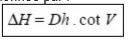surveying indirect leveling ₪ 2. Case where the point is inaccessible

# surveying indirect leveling ₪ 2. Case where the point is inaccessibleWe seek to know the altitude of an inaccessible point C knowing only the altitude of close point which will serve as a reference. If we can measure the horizontal distance AC, we can calculate the height difference from to by measuring the angle V read from to C and the height of the axis of the trunnions ht. The altitude
is given by :A solution to get the horizontal distance Dh is to create a base AB by adding a second point B, to measure  the horizontal angles CBA and CAB.
This ^makesb it possible to solve the triangle ABC and therefore to calculaate the horizontal distances AC and BC. The altitude of C is then:We can also calculate the altitude of twice: :
a firstg time from A
And a second from B and this control the result.
For this, it is necessary to determine the  ht à chaque station.